# Eliminate The noise mixed in with the HX711 readings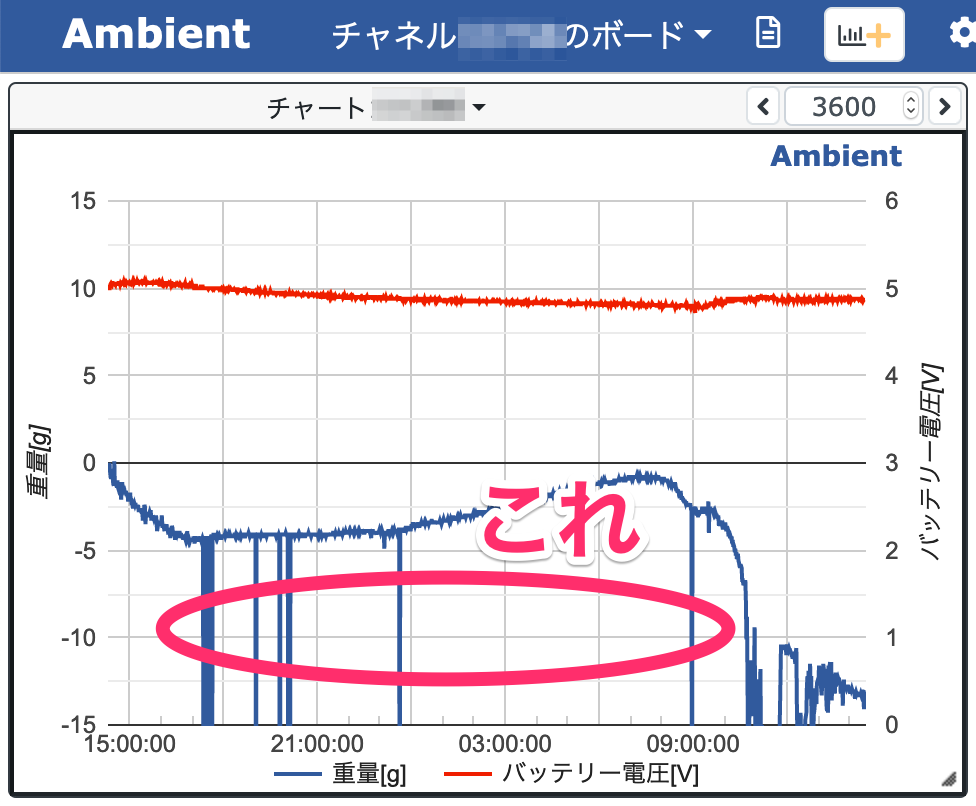I am checking the stability of a scale made with HX711 and a strain gauge, and abnormal values are measured quite often.

I am measuring the weight of the scale without placing anything on it, and sending the measurements to Ambient, a cloud service, to monitor the long-term stability of the scale, but abnormal values are occurring frequently.

## Large anomalies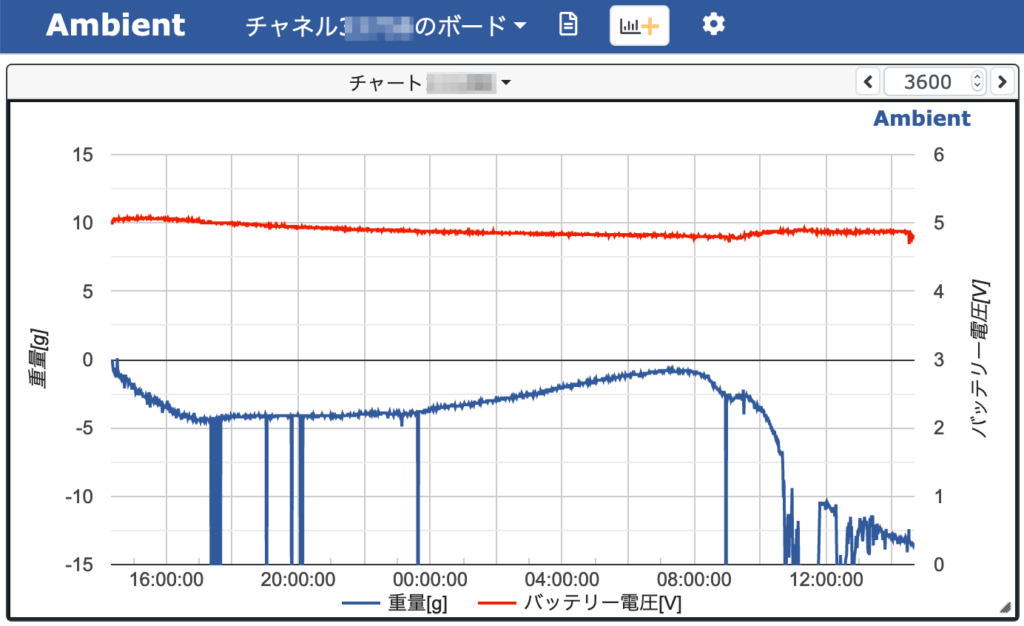This is the change in the weight measurement from the start of measurement until one day has passed. Blue is the measured weight value. Red is the battery voltage.

Since I don't put anything on the scale, it is correct that it will remain at 0g, but I can see that it fluctuates quite a bit throughout the day.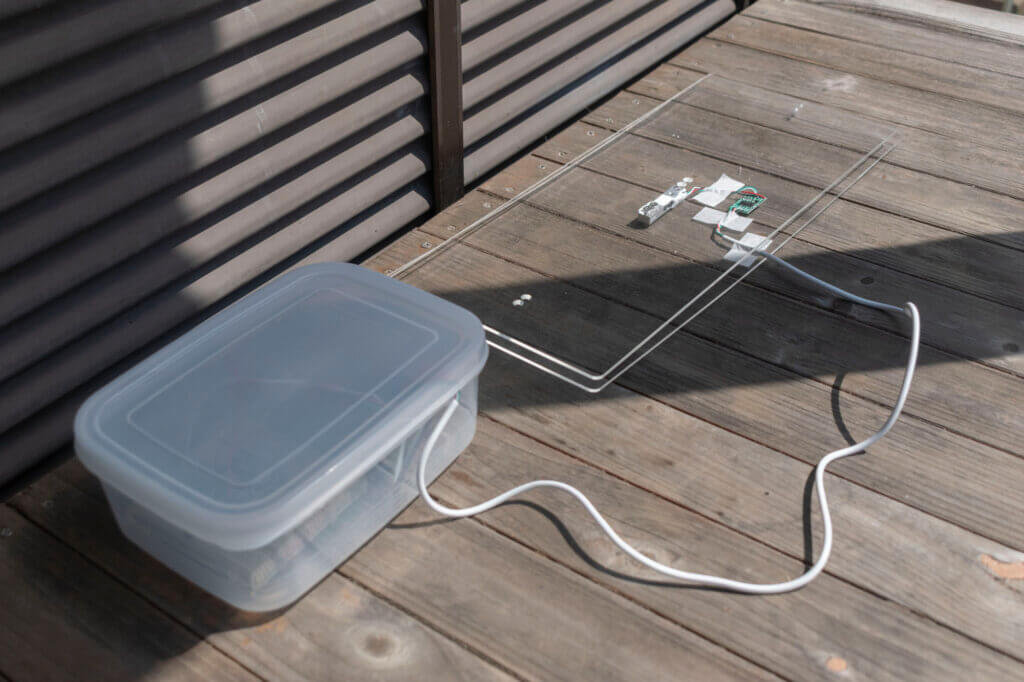Since the scale is left in a place where it is exposed to sunlight, it is likely that the temperature changes as it is exposed to sunlight or shade, and the weight being measured also changes.

Among such gradual changes in weight, we can see that occasionally, large anomalies of -15g or more are measured. This is a major obstacle in determining the presence or absence of a few grams of mail.

So, I searched for a way to somehow eliminate the abnormal values and noise in the HX711 readings.

The flow of the process so far is as follows.

• Wake up from DeepSleep
• Initialization
• Weight measurement
• Send value to Abient
• DeepSleep

The HX711 is designed to send the parameters for the next measurement when it measures the weight and reads the result. Looking at the HX711 library used in this project, I found that no parameters were sent during initialization. Therefore, the parameters of the HX711 may not be set correctly.

So I programmed...

```  long value = scale.get_units(1);  //dummy measurement
value = scale.get_units(20);
```

As above, I tried to make one dummy measurement before the main measurement.

And then...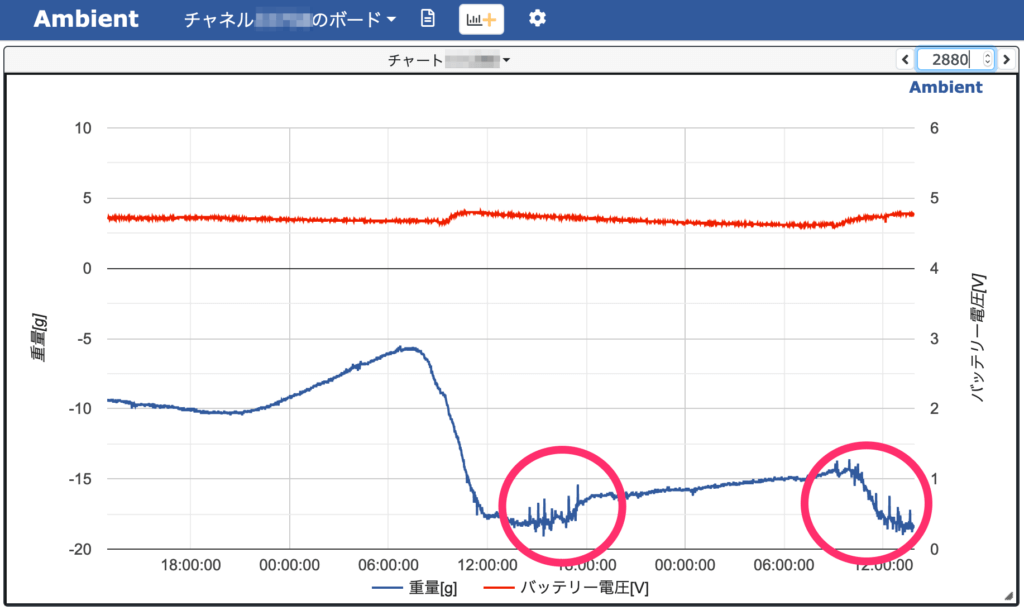Large anomalous values are no longer measured. It seems that the HX711 was not initialized correctly and the correct value could not be measured in some cases.

However, this time, if you look at the graph, you can see that a small noise continues for a while around 12:00.

Now I will try to counteract this small noise.

## Increase the number of measurements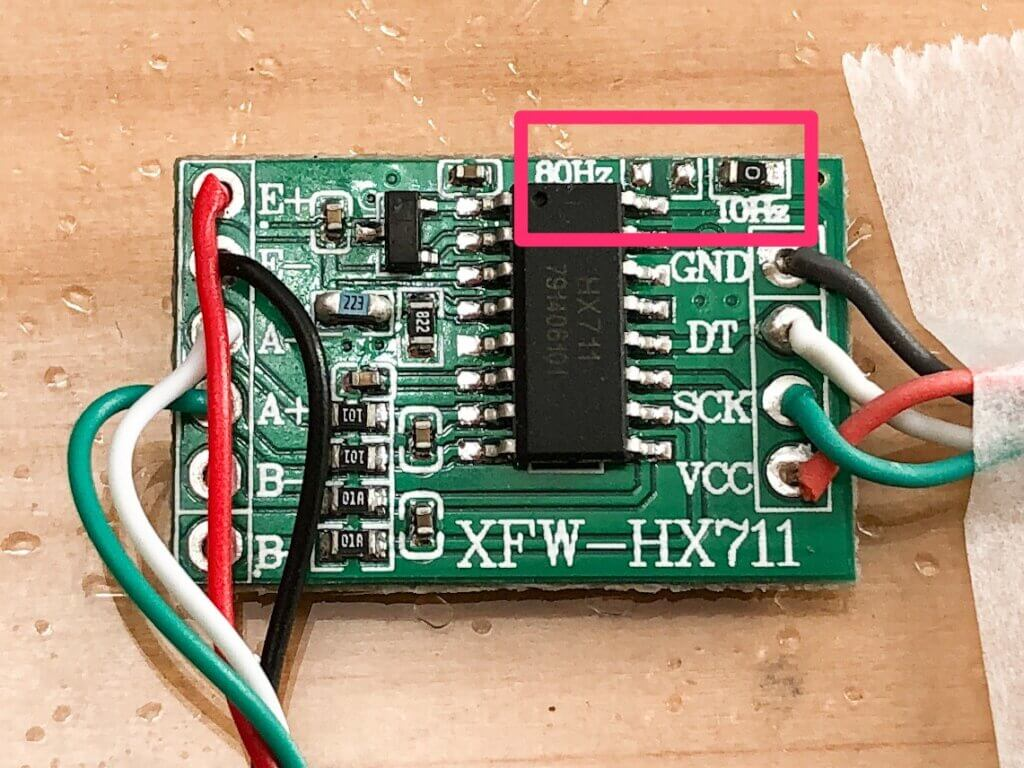The HX711 has two modes, one for measuring at 10 Hz and the other for measuring at 80 Hz. So I will try to see if the noise can be reduced by shortening the measurement cycle and increasing the average number of measurements by taking many measurements.

In the upper right corner of the module, where it says 10Hz, there is a 0Ω mounted, and to the left of that is a pad that says 80Hz and is not mounted. By changing this 0Ω resistor from 10Hz to 80Hz, the measurement frequency can be changed to 80Hz.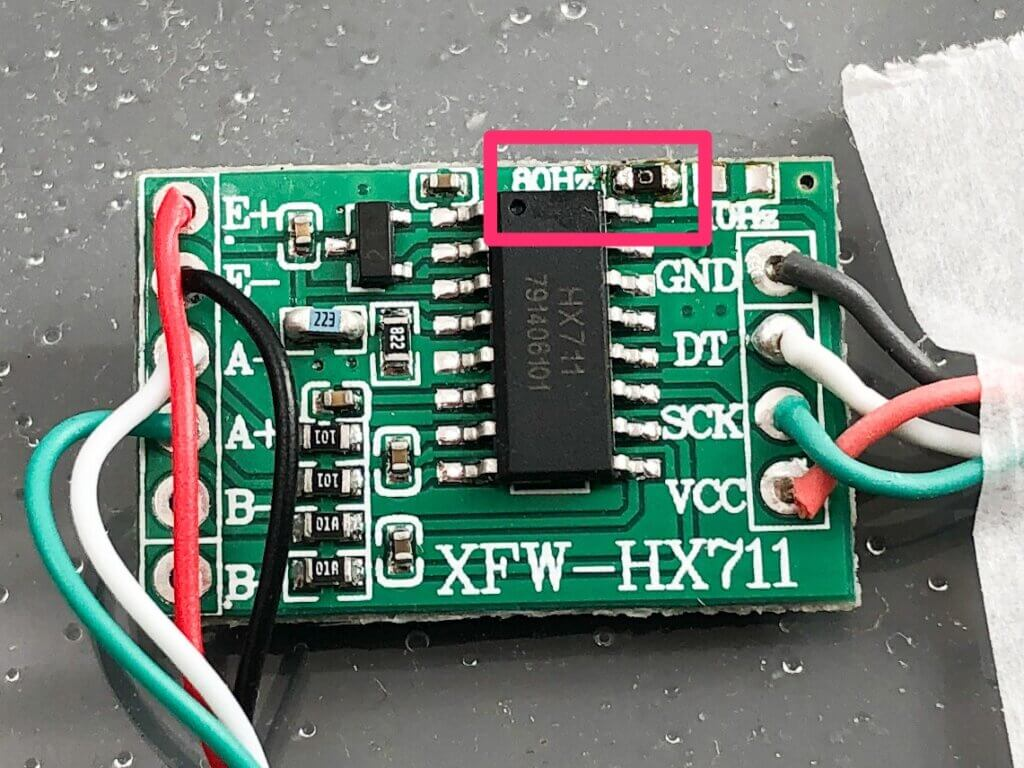I set it to 80Hz mode.

I set it to 80Hz mode, but the measurement cycle only became about four times faster. Is it because the time for communication with the HX711 is not shortened? So, I increased the number of times I averaged from 5 to 20, and took data throughout the day.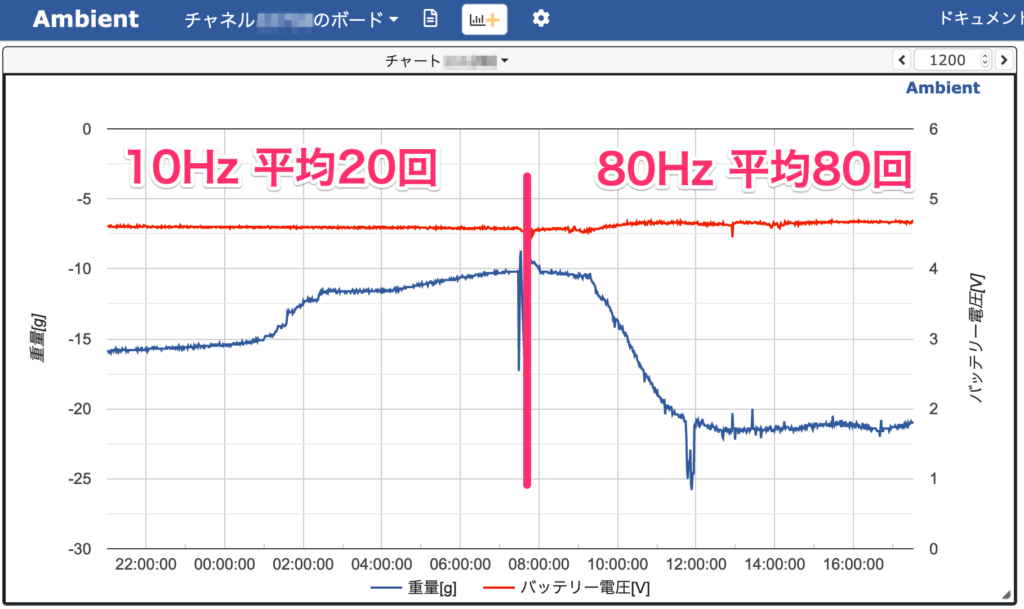The graphs above are before and after the modification. The small noises were smaller than before, but they did not disappear. It seems that even if we increase the number of averages, the small outliers cannot disappear!

## Eliminate outliers

Increasing the number of averages did not remove the noise, so we came up with a way to remove the outliers.

Assume the following.

• At least two normal values obtained after three measurements
• Abnormal values are greater than or less than normal

So, the program should look like this.

• Measure the weight three times
• Sort the results of the three times in order of heaviness
• Exclude the heaviest value and the lightest value because they may contain anomalies.
(Adopt the middle weight of the three)

Here is the actual program.

```  //Measurment Weight
long value = scale.get_units(1);  //dummy measurment
double weightTemp;
value = scale.get_units(8);
weightTemp = (double)(value) * 0.1;
value = scale.get_units(8);
weightTemp = (double)(value) * 0.1;
value = scale.get_units(8);
weightTemp = (double)(value) * 0.1;

//sort
double weight;
if( weightTemp < weightTemp )
{
weight = weightTemp;
weightTemp = weightTemp;
weightTemp = weight;
}
if( weightTemp < weightTemp )
{
weight = weightTemp;
weightTemp = weightTemp;
weightTemp = weight;
}
if( weightTemp < weightTemp )
{
weight = weightTemp;
weightTemp = weightTemp;
weightTemp = weight;
}
weight = weightTemp; //select a center value
```

Here are the measurement results after changing to this program.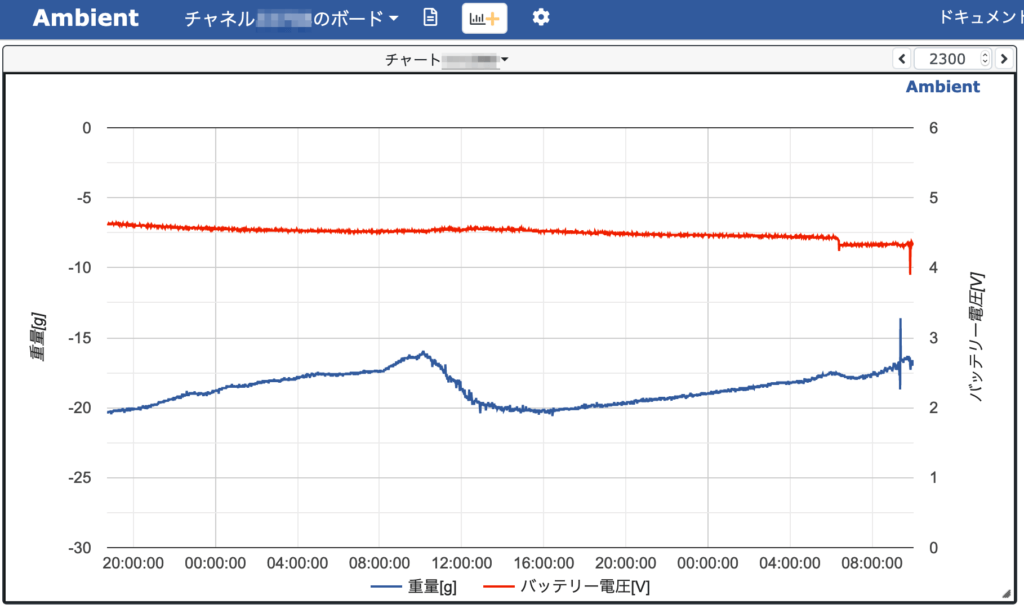The small noise is gone and the waveform is now clean.

There is still a small, small noise at about 12:00, but I think the measurement error has been reduced to about 1g.

## What we learned from long-term measurements

What I found out after measuring continuously throughout 7 days is that the weight changes depending on the day and time. Some days the change was as much as 12 grams in one day. For this reason, it is not possible to determine that a piece of mail has been delivered because it weighs a certain number of grams. It may be necessary to compare the difference, such as how many grams have been increased from one previous measurement, to determine that the mail has arrived.